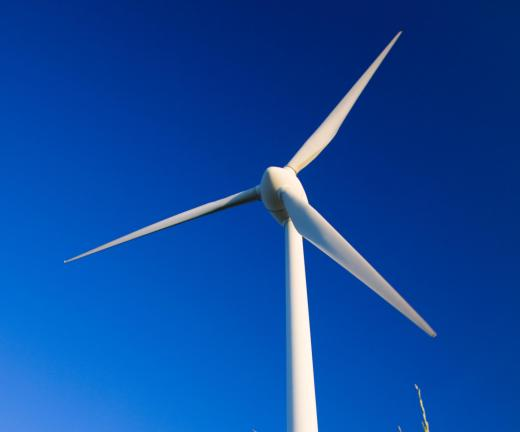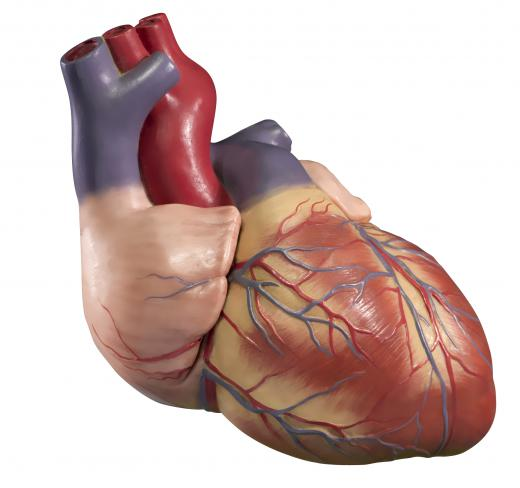Science
Fact Checked

# What are the Different Mechanical Energy Examples?

Daniel Liden
Daniel Liden

Mechanical energy is energy that comes from any mechanical system; it is usually involved with physical motion and work. Examples include falling weights, the Earth's rotation, pendulums and flowing water. Mechanical energy is the sum of two forms of energy: kinetic energy and potential energy. Potential energy is energy that comes from the position of an object or the configuration of parts of a system; it has yet to be converted to any specific kind of energy. Kinetic energy is energy that exists because of the motion of an object or of the objects in a system.

Many students conduct experiments in high school or college to demonstrate various mechanical energy examples. Falling weights or rotating objects contain mechanical energy, as do pendulums; these are commonly studied in introductory physics courses. Some students also learn about the conversion of energy types, which can be clearly seen in a simple electric motor, which converts electrical energy into mechanical energy.Windmills harness the energy of the wind and convert it into mechanical energy and then to electrical energy.

There are many mechanical energy examples that exist in nature and in the various inventions of mankind. Examples in nature are commonplace. On a very large scale, the motion and rotation of the Earth and other other celestial bodies is mechanical energy. On a smaller scale, the motion of water as it flows in a river or stream is also an example of mechanical energy. Even such simple processes as the falling of stones or the movement of dust in the wind are all a type of mechanical energy.The human heart uses mechanical energy to pump blood throughout a person's body.

Humans have devised many ways to generate and make use of mechanical energy, so the machinery designed by humans presents many different mechanical energy examples. Motors present a simple example; they convert one form of energy, usually chemical energy, that is released through combustion into mechanical energy that allows for some form of motion. This is most commonly seen in automobiles, which are primarily propelled by internal combustion engines.

Mechanical energy can also be transformed into different forms of energy; some means of producing electrical energy present good mechanical energy examples. Windmills, for example, convert mechanical energy into electrical energy. Mechanical energy is at work when the wind turns the blades of the windmill; this energy is converted into electrical energy.

Even living creatures are full of mechanical energy examples: birds fly, people walk, and fish swim. The human heart uses mechanical energy to pump blood throughout the body. Such energy is even used in simple acts such as lifting objects or blinking.

## You might also LikeFiorite

@ GiraffeEars- You are asking a question that relates to the laws of thermodynamics. While the law of conservation of energy states that all energy must be accounted for, the second law of thermodynamics attests to the chaotic nature of energy. The potential energy transferred from one source to the next will always be less than the original source. Energy can change, but cannot return to its original state in whole. The chemical energy that from the gasoline not only becomes mechanical energy, it becomes heat, and work on other components as friction, and potentially static electricity. The efficiency of an engine depends on the amount of energy transferred from a given amount of chemical potential energy to mechanical kinetic energy.

GiraffeEars

This was an informative article on examples of mechanical energy. I do have a question though. If a motor simply transfers chemical energy into mechanical energy, then why are they sometimes so inefficient? You can have two motors with the same displacement, but they get completely different fuel economies. Wouldn't the transfer of energy be the same for motors of the same displacement?